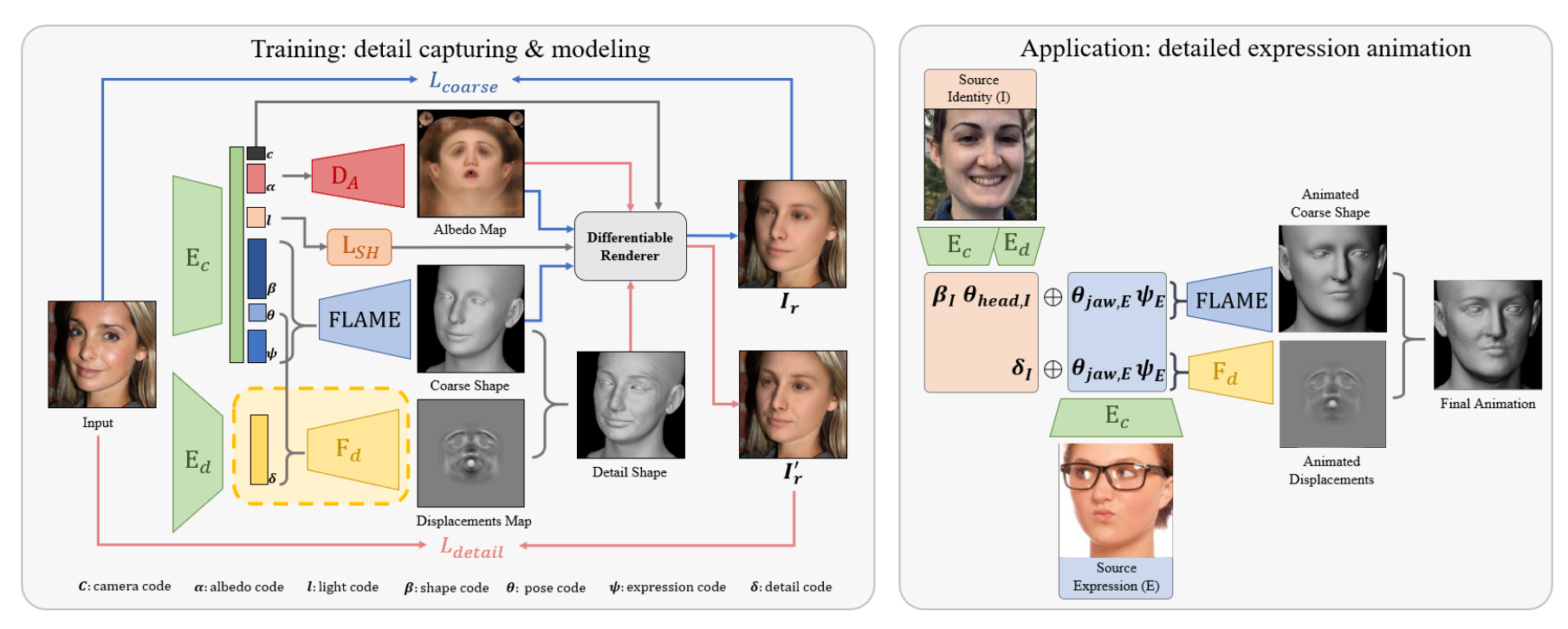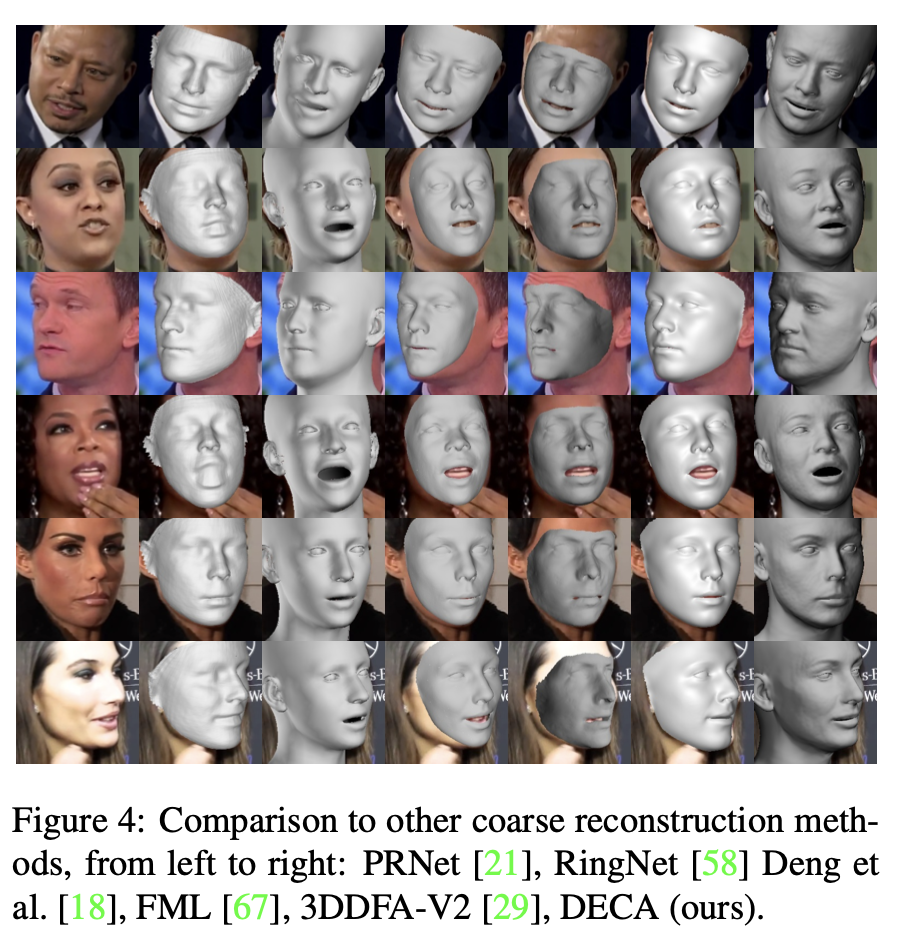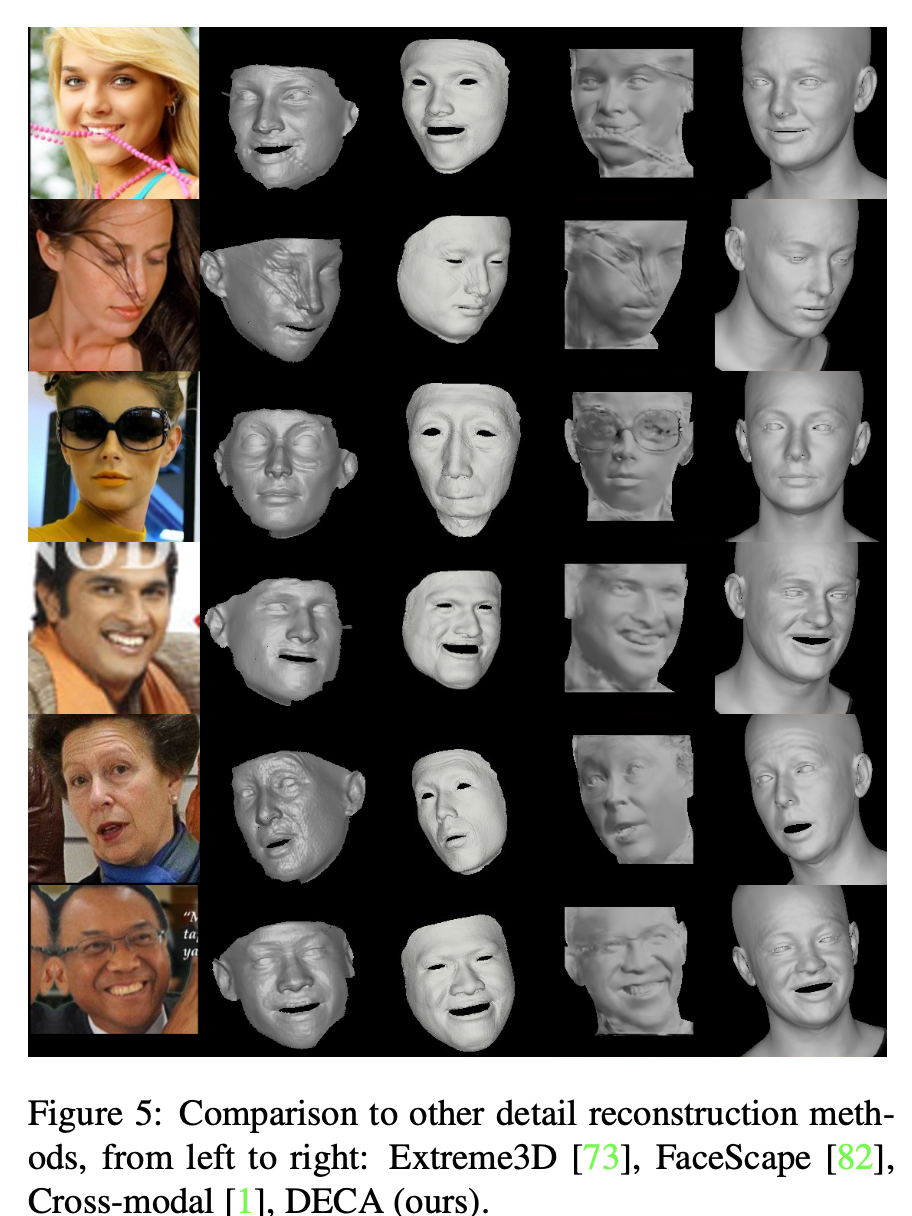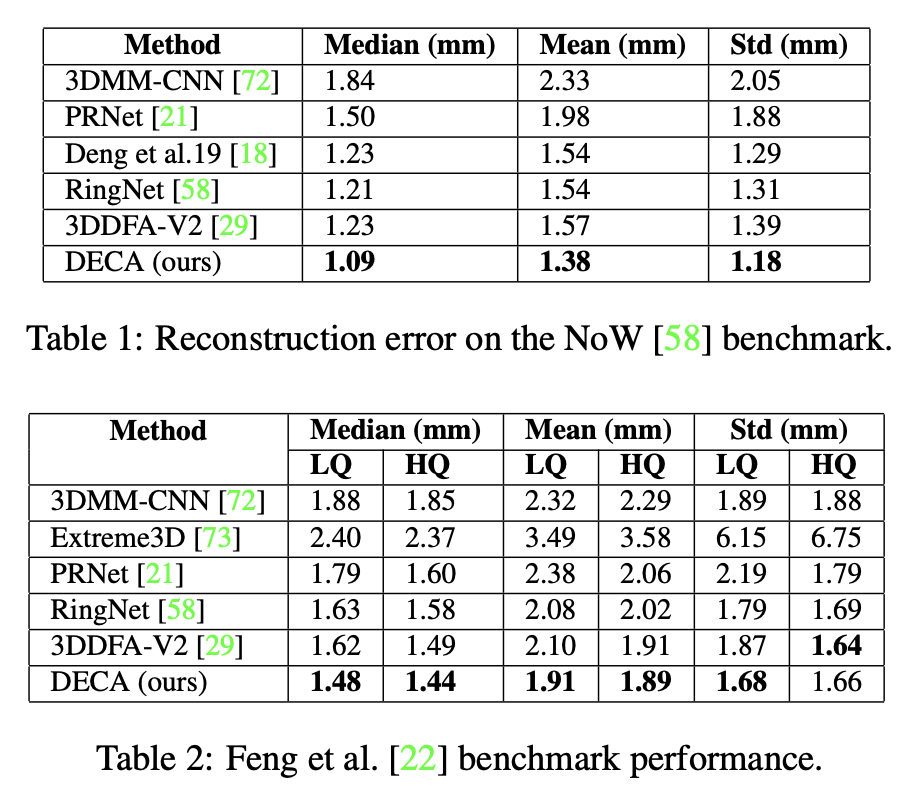# Learning an Animatable Detailed 3D Face Model from In-The-Wild Images

This is my reading note for paper Learning an Animatable Detailed 3D Face Model from In-The-Wild Images and code is available in DECA. The paper proposed method for producing animatable detailed 3D face model from uncontrolled image datasets. For uncontrolled, it means no control light settings and camera view angles are required; however it does require the dataset contains multiple images for each subjets.

There are two major contributions/novelties of this paper:

• reconstruct the 3D face with coarse component and then detail component;
• propose detail-consistency loss to seperate identity specific detail and expression specific details.

# Related Work

The 3D face reconstruction methods can be roughly divided into the follow groups:

• Coarse reconstruction: Many monocular 3D face reconstruction methods follow Vetter and Blanz  by estimating coefficients of pre-computed statistical models in an analysis-by-synthesis fashion.
• Instead of capturing high-frequency geometric details, some methods reconstruct coarse facial geometry along with high-fidelity textures [24, 57, 65, 81]. As this “bakes” shading details into the texture, lighting changes do not affect these details.
• Detail reconstruction: Most regression-based approaches follow a similar approach by first reconstructing the parameters of a statistical face model to obtain a coarse shape, followed by a refinement step to capture localized details.
• Animatable detail reconstruction: Chaudhuri et al.  learn identity and expression corrective blendshapes with dynamic (expression-dependent) albedo maps . They model geometric details as part of the albedo map, and therefore, the shading of these details does not adapt with varying lighting. This results in unrealistic renderings.

# Proposed Method

The key idea of DECA is grounded in the observation that an individual’s face shows different details (i.e. wrinkles), depending on their facial expressions but that other properties of their shape remain unchanged. Consequently, facial details should be separated into static person-specific details and dynamic expression-dependent details such as wrinkles.

DECA learns an expression-conditioned detail model to infer facial details from both the person-specific detail latent space and the expression space. To do this detail-consistency loss is used, which enforces that exchanging personal specific detail code for images from the same identity should result in low reconstruction error.

DECA is illustrated as below: $$E_c$$ is coarse encoder, $$E_d$$ is the detail encoder and $$F_d$$ is the detail decoder. Both encoders uses ResNet50.## Coarse Reconstruction

We first learn a coarse reconstruction (i.e. in FLAME’s model space) in an analysis-by-synthesis way: given a 2D image I as input, we encode the image to a latent code, decode this to synthesize a 2D image, and minimize the difference between the synthesized image and the input. More specifically, the coarse geometry uses the first 100 FLAME shape parameters $$\beta$$, 50 expression parameters $$\psi$$, and 50 albedo parameters $$\alpha$$. In total, $$E_c$$ predicts a 236 dimensional latent code.

For each item in the training data, we have a 2D face image, identity label and 68 facial landmarks. The coarse encoder is trained with following loss:

• Landmark re-projection loss;
• Eye closure loss: The eye closure loss computes the relative offset of landmarks on the upper and lower eyelid, and measures the difference to the offset of the corresponding landmarks in the FLAME’s surface projected into the image.
• Photometric loss: The photometric loss computes the error between the input image and the rendering for face skin region only;
• Identity loss: measures the cosine similarity between the two embeddings computed by a face recognition network.
• Shape consistency loss: Given two images of the same subject , the coarse encoder $$E_c$$ should output the same shape parameters (i.e. $$\beta_i=\beta_j$$). We propose a different strategy by replacing $$\beta_i$$ with $$\beta_j$$ while keeping all other parameters unchanged. Given that $$\beta_i$$ and $$\beta_j$$ represent the same subject, this new set of parameters must reconstruct $$I_i$$ well.
• Regularization: l2 norm applied to shape, expression and albedo.

## Detail Reconstruction

The detail reconstruction aims at augmenting the coarse FLAME geometry with a detailed UV displacement map $$D\in\left[-0.01,0.01\right]^{d\times d}$$. Similar to the coarse re- construction, we train an encoder $$E_d$$ (with the same architecture as $$E_c$$) to encode I to a 128-dimensional latent code $$\delta$$, representing subject-specific details. The latent code $$\delta$$ is then concatenated with FLAME’s expression $$\psi$$ and jaw pose parameters $$\theta_{jaw}$$, and decoded by $$F_d$$ to D. Then final mesh $$M_{UV}^{D}$$ combining detail displacement D and coarse mesh $$M_{UV}$$ ($$N_{UV}$$ is the surface normal for coarse mesh) can be written as:

$M_{UV}^{D}=M_{UV}+D\odot N_{UV}$

The detail encoder and decode is trained with the following loss:

• Detail photometric losses
• ID-MRF loss: We add an Implicit Diversified Markov Ran- dom Fields (ID-MRF) loss to reconstruct geometric details. This is applied to intemediate layers of encoder as well.
• Soft symmetry loss: To add robustness to occlusions, we add a soft symmetry loss to regularize non-visible face parts, which is $$\ell_{sym}=\lVert V_{UV}-\odot(D-\mbox{flip}(D)\rVert_1$$
• Detail regularization: l1 norm on detail code to reduce noise.

## Detail Disentanglement

One of the most important novelties of this paper is detail-consistency loss to seperate identity specific detail and expression specific details. This is achieved by for rendered detail image, exchanging the detail codes between two images of the same subject should have no effect on the rendered image.

# Experiment Result

Visual comparisons of coarse reconstructions:Visual comparisons of detail reconstructions.Quantiative results:Written on April 18, 2021